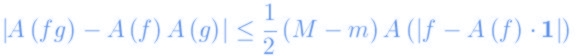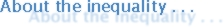Home Research Collaborations  & Co-operations Editorship & Service Miscellaneous RGMIA JIPAM AJMAA

## Professor S.S. Dragomir

### Pure Mathematics

• Classical Mathematical Analysis

• Convex Functions

• Best Approximation

• Numerical Integration

• Geometry of Banach Spaces

• Operator Theory

• Variational Methods

• Volterra Integral Equations

• Qualitative Theory of Differential Equations

### Applied Mathematics

• Theory and Coding

• Guessing Theory

• Numerical Methods for Differential Equations

• Numerical Methods for PDE's

• Game Theory

• Kolmogorov Complexity